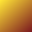#X = X + 1

The value of variables may change over time as a computer program runs.

When we say something like X = X+1 we are acknowledging the advance of time.

A mathematician might say X' = X+1 so as to preserve the traditional meaning of equality.

Some computer languages use symbols like := or <- in place of = so as to avoid the conflict with mathematical notation.

Some computer languages let you bind a value to a name only once. In such a language you could say X = 2+1 or Y = X+1 but not X = X+1

The value of a variable at some point in time is called its state. The value of all variables taken together (including some hidden variables) is the state of the program.